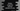# Python program to swap the first and the last element of a list# Python program to swap the first and the last element of a list :

Python list is one of the most frequently used datatypes. A list is used to hold a group of elements. These elements are placed inside a square bracket separated by commas. Python list can hold items of different datatypes e.g. a list can have items of type_ ‘integer’, ‘float’, ‘string’_ etc.

Unlike strings, we can change the items of a list. The square bracket is used to access an element of a list. We need to pass the index of the element for that. For example, ‘mylist[i]’_ will return the item on index_ ‘i’_ for the list ‘mylist’. The index starts from ‘0’, i.e. ‘0’_ is the index of the first element,_ ‘1’_ is the index of the second element etc.

In this python programming tutorial, we will learn how to swap the first and the last element of a list. The list is already given to the program. It will swap the elements and print out the new list.

## Python program :

If you know how to swap two numbers in python or any other language, this program uses the same logic. Create one temporary variable to hold the last value, assign the first element value to the last element and assign the temporary element value to the first element. That’s it.

Let’s take a look at the program :

``````#1
user_list = [1,2,5,4,6,7,8]

#2
temp = user_list[len(user_list) - 1]

#3
user_list[len(user_list) - 1] = user_list

#4
user_list = temp

#5
print(user_list)``````

(You can also download the source code from here) :

## Explanation :

The commented numbers in the above program denote the step numbers below :

1. userlist_ is the given list holding 7 numbers. Our program will swap the first and the last element of this list. This is a predefined list and we are using only numbers in this list. You can also take the list elements as input from the user. This list can also hold different datatypes.
2. Get the last element of the list. This is the element of index position_ (length of the list - 1). The index starts from _‘0’. The index of the 1st element is_ ‘0’, the _2nd element is_ ‘1’_ etc. So, the index of the last element is (length - 1). For calculating the list length, we are using len() method. This method takes the list as its argument and returns its length. Assign the value of the last element to a temporary variable ‘temp’. In this step, ‘temp’ is holding the last element’s value.
3. Assign the value of the first element of the list to the last element. We are assigning the index ‘0’ element or the first element to the index ‘len(userlist) - 1’_ element or the last element._ ’=’_ operator is used for the assignment.
4. Now, set the value stored in the temporary variable to the first element of the list.
5. Finally, print out the list.

### Output :

It will print the below output :

``[8, 2, 5, 4, 6, 7, 1]``

### Conclusion :

In this tutorial, we have learned how to swap the first and the last element of a list. Similar to this program, we can swap any two elements of a list in python easily. Go through the program and drop one comment below if you have any queries.